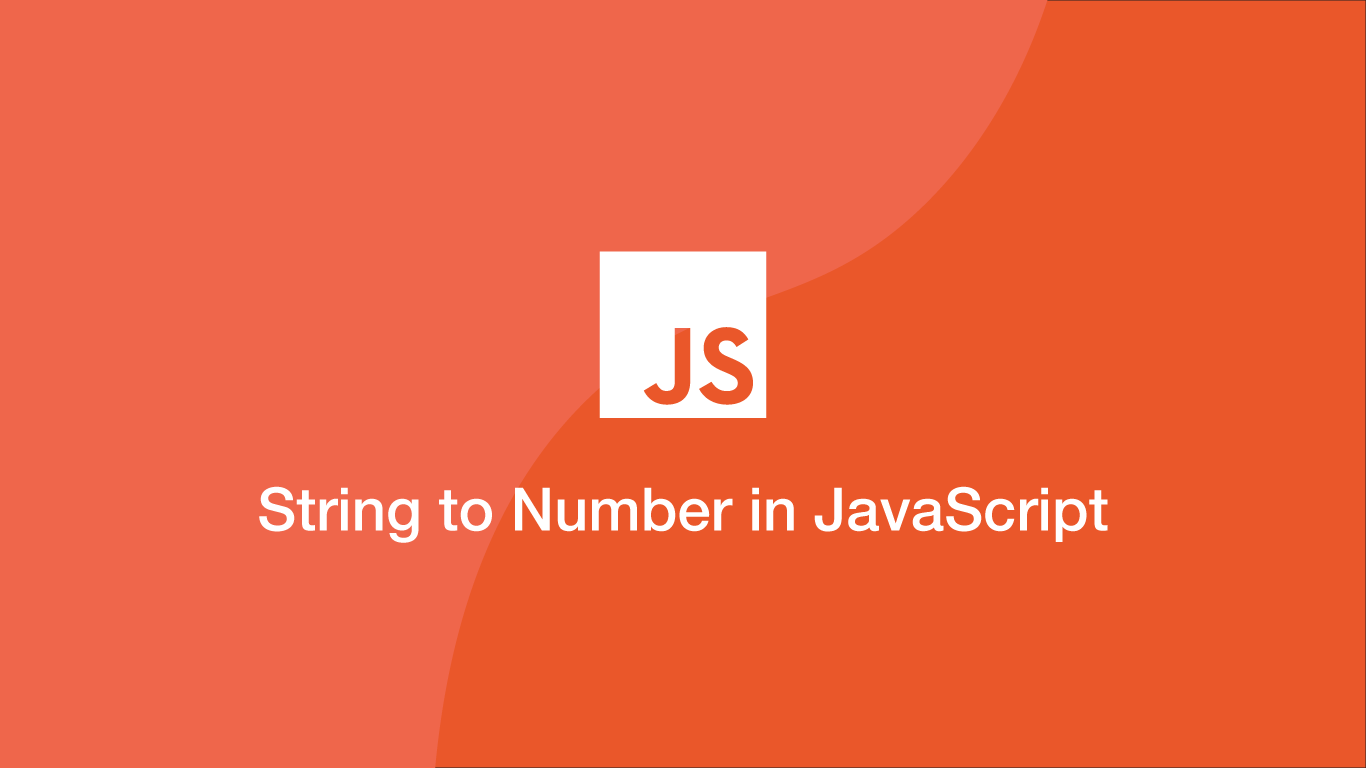# How to Convert a String to a Number in JavaScriptConverting a string to a number is a fundamental aspect of programming in JavaScript or any other major programming language for that matter.

Fortunately, JavaScript provides several options for converting strings into numbers. In this article, we will go through some of the ways we can achieve this.

## The Number() Constructor

The easiest and most readable way to convert a string to a number is to pass it into the JavaScript `Number` constructor. Just pass the string as the first argument in `Number` function.

``````var string = '123';
var num = Number(string);
``````
``````123
``````

Note - `Number` removes decimal places and will give a `NaN` error if any other separators are included in the string to be converted.

``````Number('20,000') //NaN
Number('20.00') //20
``````

## .parseInt()

`parseInt` works just like `Number`, except we can pass a second argument to convert the string into non-decimal numerical systems such as binary. `parseInt` will also treat non `.` (dot) separators as a decimal place also.

``````parseInt('222');
``````
``````222
``````
``````parseInt('222.222'); // decimal place will be removed
``````
``````222
``````
``````parseInt('222kk222'); // everything after first non number is cut
``````
``````222
``````
``````parseInt("0xF", 16); // convert hexadecimal
``````
``````15
``````
``````parseInt('s123'); // strings starting with non-numerical value will return NaN
``````
``````NaN
``````

## .parseFloat()

`parseFloat` will preserve floating-point numbers as they are converted from strings.

``````parseFloat('222');
``````
``````222
``````
``````parseFloat('22.02');
``````
``````22.02
``````
``````parseFloat('22.00'); // decimal place is removed from floating points consisting of only zeros.
``````
``````22
``````

Just like with `parseInt`, everything after a non-numerical value is removed and strings starting with a non-numerical value will be returned as `NaN`.

``````parseFloat('222kk222'); // everything after first non number is cut
``````
``````222
``````
``````parseFloat('kk222');
``````
``````NaN
``````

## Math.floor()

`Math.floor` will accept strings making it a convenient way to round-down floating point numerical strings into number objects in JavaScript. You will need to make sure the string has no non-numerical values in it beforehand though.

``````Math.floor('22.72');
``````
``````22
``````

If there are any non-numerical characters in the string it will output `NaN`.

``````Math.floor('22.72');
``````
``````NaN
``````

## Math.ceil()

`Math.ceil` will also accept strings and convert them into number objects, making it a clean way to simultaneously convert a numerical string and round it up. It has the same behaviour as `Math.floor` in terms of what numerical strings it will accept.

``````Math.ceil('22.22');
``````
``````23
``````

## Multiply by 1

You can also simply times the numerical string by 1 and JavaScript will turn it into a number object. Of course, the numerical string must be formatted correctly before multiplying it.

``````var string = '22.72';
string * 1
``````
``````22.72
``````

## Conclusion

You now know multiple ways in which you can change a string to a number object in JavaScript and how each function behaves.

#### Related Tutorials### parseString in JavaScript

March 16, 2021### How to use the parseInt() Function in JavaScript

July 15, 2021### How to Compare Values in JavaScript

August 26, 2020### Convert to Lowercase or Uppercase in JavaScript

November 09, 2020### How to Parse JSON Data in Python (Read and Write)

September 18, 2020July 15, 2020